# SSAT Upper Level Math : How to find the equation of a circle

## Example Questions

2 Next →

### Example Question #11 : How To Find The Equation Of A Circle

A square on the coordinate plane has as its vertices the points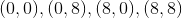. Give the equation of a circle inscribed in the square.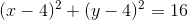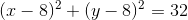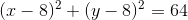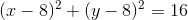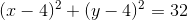Explanation:

Below is the figure with the circle and square in question: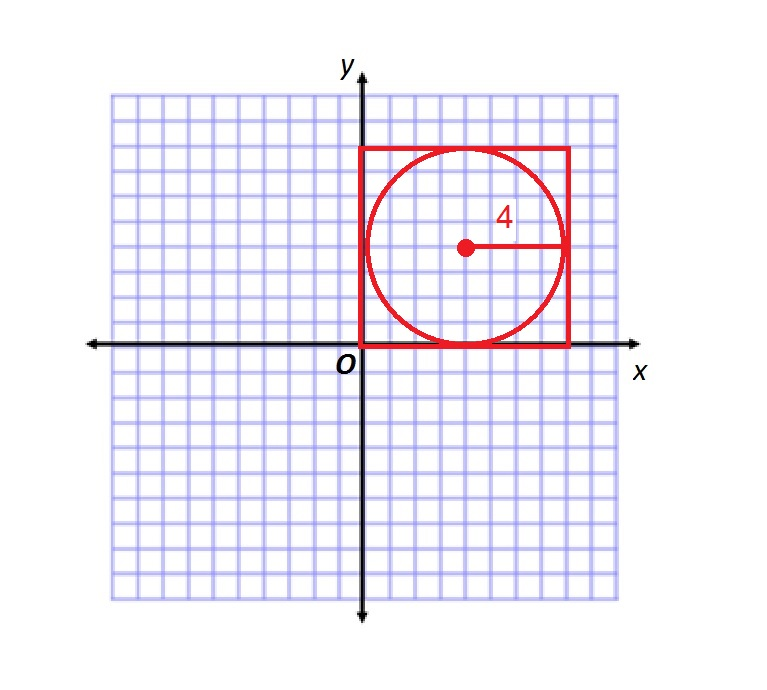The center of the inscribed circle coincides with that of the square, which is the point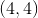. Its diameter is equal to the sidelength of the square, which is 8, so, consequently, its radius is half this, or 4. Therefore, in the standard form of the equation,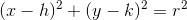,

substitute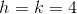and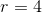.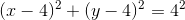### Example Question #12 : How To Find The Equation Of A Circle

Give the area of the circle on the coordinate plane whose equation is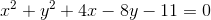.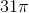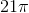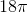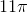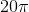Explanation:

The standard form of the equation of a circle iswhereis the radius of the circle.

We can rewrite the equation we are given, which is in general form, in this standard form as follows: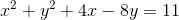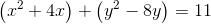Complete the squares. Since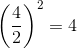and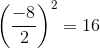, we do this as follows: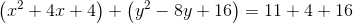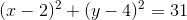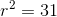, and the area of the circle is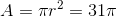### Example Question #13 : How To Find The Equation Of A Circle

Which of the following is the equation of a circle with center at the origin and area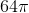?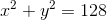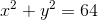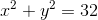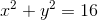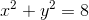Explanation:

The standard form of the equation of a circle is,

where the center is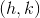and the radius is.

The center of the circle is the origin, so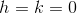, and the equation is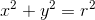for some.

The area of the circle is, so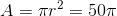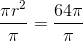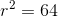We need go no further; we can substitute to get the equation.

### Example Question #14 : How To Find The Equation Of A Circle

Which of the following is the equation of a circle with center at the origin and circumference?None of the other responses gives the correct answer.None of the other responses gives the correct answer.

Explanation:

The standard form of the equation of a circle is,

where the center isand the radius isThe center of the circle is the origin, so.

The equation will befor some.

The circumference of the circle is, so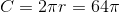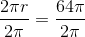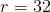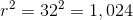The equation is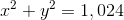, which is not among the responses.

2 Next →